Graphs of: y = sin(x) and y = cos(x) MathBitsNotebook.com Terms of Use   Contact Person: Donna RobertsGraphs of trigonometric functions can be produced in degrees or in radians.
The graphs appearing here will be done in radians.When hand-drawing the graphs of sine and cosine, draw vertical dotted lines at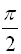intervals to remind you of the connection to the four quadrants from the unit circle and to keep your graphs accurate.  (Such dotted lines will resemble the dashed vertical grid lines in the graphs below.)

The sine and cosine functions take on y-values between -1 and +1.Sine Function:   y = sin x
y = sin x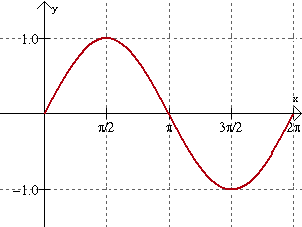called a "wave" because of its rolling wave-like
appearance (also referred to as oscillating)
amplitude: 1  (height)
period: 2π  (length of one cycle)
frequency:  1cycle in 2π radians [or 1/(2π)]
domain: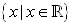range: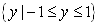At x = 0, the sine wave is on the shoreline! (meaning the y-value is equal to zero)Cosine Function:   y = cos x
y = cos x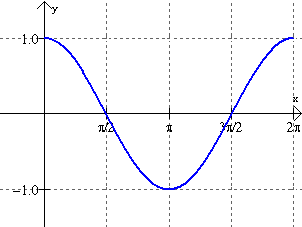called a "wave" because of its rolling wave-like
appearance
amplitude: 1
period: 2π

frequency:  1 cycle in 2π radians [or 1/(2π )]

domain:range:At x = 0, the cosine wave breaks off the cliff! (meaning the y-value is equal to one)

Remember:
The cosine curve is really the exact same graph as the sine curve shifted 90º, or π/2 radians, to the left!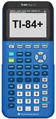How to use your graphing calculator for graphing trig functions. click here.